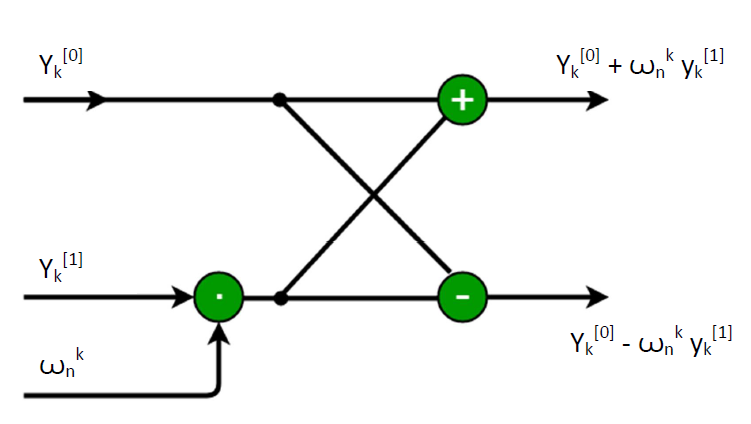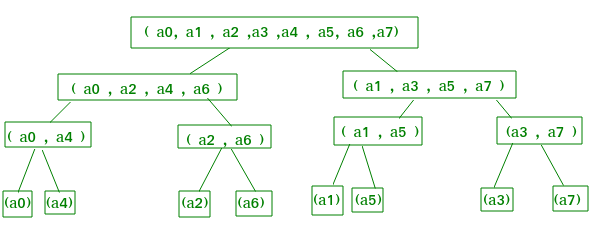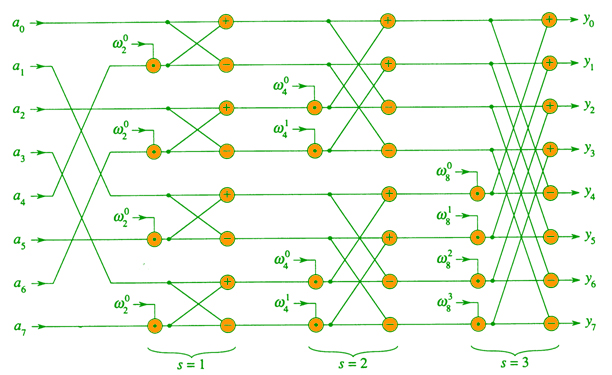GeeksforGeeks App
Open AppBrowser
Continue

# Iterative Fast Fourier Transformation for polynomial multiplication

Given two polynomials, A(x) and B(x), find the product C(x) = A(x)*B(x). In the previous post, we discussed the recursive approach to solve this problem which has O(nlogn) complexity.
Examples:

Input :
A[] = {9, -10, 7, 6}
B[] = {-5, 4, 0, -2}
Output :
C(x) =In real-life applications such as signal processing, speed matters a lot, this article examines an efficient FFT implementation. This article focuses on the iterative version of the FFT algorithm that runs in O(nlogn) time but can have a lower constant hidden than the recursive version plus it saves the recursion stack space.
Pre-requisite: recursive algorithm of FFT.
Recall the recursive-FFT pseudo code from previous post, in the for loop evaluation ofis calculated twice. We can change the loop to compute it only once, storing it in a temporary variable t. So, it becomes,
for k0 to n/2 – 1
do tThe operation in this loop, multiplying the twiddle factor w =by, storing the product into t, and adding and subtracting t from, is known as a butterfly operation.
Pictorially, this is what a butterfly operation looks like:Let us take for n=8 and proceed with the formation of the iterative FFT algorithm. Looking at the recursion tree above, we find that if we arrange the elements of the initial coefficient vector into the order in which they appear in the leaves, we could trace the execution of the Recursive-FFT procedure, but bottom-up instead of top-down. First, we take the elements in pairs, compute the DFT of each pair using one butterfly operation, and replace the pair with its DFT. The vector then holds n/2 2-element DFTs. Next, we take these n/2 DFTs in pairs and compute the DFT of the four-vector elements they come from by executing two butterfly operations, replacing two 2-element DFTs with one 4-element DFT. The vector then holds n/4 4-element DFTs. We continue in this manner until the vector holds two (n/2) element DFTs, which we combine using n/2 butterfly operations into the final n-element DFT.To turn this bottom-up approach into code, we use an array A[0…n] that initially holds the elements of the input vector a in the order in which they appear in the leaves of the tree. Because we have to combine DFT so n each level of the tree, we introduce a variable s to count the levels, ranging from 1 (at the bottom, when we are combining pairs to form 2-element DFTs) to lgn (at the top, when we are combining two n/2 element DFTs to produce the final result). The algorithm therefore is:

1. for s=1 to lgn
2.     do for k=0 to n-1 by3.           do combine the two-element DFTs in                A[k...k+-1] and A[k+...k+-1]                into one 2s-element DFT in A[k...k+-1]

Now for generating the code, we arrange the coefficient vector elements in the order of leaves. Example- The order in leaves 0, 4, 2, 6, 1, 5, 3, 7 is a bit reversal of the indices. Start with 000, 001, 010, 011, 100, 101, 110, 111 and reverse the bits of each binary number to obtain 000, 100, 010, 110, 001, 101, 011, 111.Pseudocode for iterative FFT :

BIT-REVERSE-COPY(a, A)
n = length [a]
for k = 0 to n-1
do A[rev(k)] = a[k]

ITERATIVE-FFT
BIT-REVERSE-COPY(a, A)
n = length(a)
for s = 1 to log n
do m==for j = 0 to m/2-1               do for k = j to n-1 by m                      do t =A[k+m/2]                         u = A[k]                         A[k] = u+t                         A[k+m/2] = u-treturn A

It will be more clear from the below parallel FFT circuit :## CPP

 // CPP program to implement iterative// fast Fourier transform.#include using namespace std; typedef complex<double> cd;const double PI = 3.1415926536; // Utility function for reversing the bits// of given index xunsigned int bitReverse(unsigned int x, int log2n){    int n = 0;    for (int i = 0; i < log2n; i++) {        n <<= 1;        n |= (x & 1);        x >>= 1;    }    return n;} // Iterative FFT function to compute the DFT// of given coefficient vectorvoid fft(vector& a, vector& A, int log2n){    int n = 4;     // bit reversal of the given array    for (unsigned int i = 0; i < n; ++i) {        int rev = bitReverse(i, log2n);        A[i] = a[rev];    }     // j is iota    const complex<double> J(0, 1);    for (int s = 1; s <= log2n; ++s) {        int m = 1 << s; // 2 power s        int m2 = m >> 1; // m2 = m/2 -1        cd w(1, 0);         // principle root of nth complex        // root of unity.        cd wm = exp(J * (PI / m2));        for (int j = 0; j < m2; ++j) {            for (int k = j; k < n; k += m) {                 // t = twiddle factor                cd t = w * A[k + m2];                cd u = A[k];                 // similar calculating y[k]                A[k] = u + t;                 // similar calculating y[k+n/2]                A[k + m2] = u - t;            }            w *= wm;        }    }} int main(){    vector a{ 1, 2, 3, 4 };    vector A(4);    fft(a, A, 2);    for (int i = 0; i < 4; ++i)        cout << A[i] << "\n";}

## Java

 import java.io.*;import java.lang.*;import java.util.*;import java.util.stream.*; public class Main {    static class Complex {        double real, imag;         public Complex(double r, double i)        {            real = r;            imag = i;        }         public Complex() { this(0, 0); }         public Complex add(Complex b)        {            return new Complex(real + b.real,                               imag + b.imag);        }         public Complex subtract(Complex b)        {            return new Complex(real - b.real,                               imag - b.imag);        }         public Complex multiply(Complex b)        {            return new Complex(                real * b.real - imag * b.imag,                real * b.imag + imag * b.real);        }         public String toString()        {            return "(" + real + ", " + imag + ")";        }    }     // Utility function for reversing the bits    // of given index x    static int bitReverse(int x, int log2n)    {        int n = 0;        for (int i = 0; i < log2n; i++) {            n <<= 1;            n |= (x & 1);            x >>= 1;        }        return n;    }     // Iterative FFT function to compute the DFT    // of given coefficient vector    static void fft(List a, List A,                    int log2n)    {        int n = 1 << log2n;         // bit reversal of the given array        for (int i = 0; i < n; ++i) {            int rev = bitReverse(i, log2n);            A.set(i, a.get(rev));        }         // j is iota        final Complex J = new Complex(0, 1);        for (int s = 1; s <= log2n; ++s) {            int m = 1 << s; // 2 power s            int m2 = m >> 1; // m2 = m/2 -1            Complex w = new Complex(1, 0);             // principle root of nth complex            // root of unity.            Complex wm                = new Complex(Math.cos(Math.PI / m2),                              Math.sin(Math.PI / m2));            for (int j = 0; j < m2; ++j) {                for (int k = j; k < n; k += m) {                     // t = twiddle factor                    Complex t = w.multiply(A.get(k + m2));                    Complex u = A.get(k);                     // similar calculating y[k]                    A.set(k, u.add(t));                     // similar calculating y[k+n/2]                    A.set(k + m2, u.subtract(t));                }                w = w.multiply(wm);            }        }    }     public static void main(String[] args)    {        List a = Arrays.asList(            new Complex(1, 0), new Complex(2, 0),            new Complex(3, 0), new Complex(4, 0));        List A = new ArrayList<>(            Arrays.asList(new Complex));        fft(a, A, 2);        for (int i = 0; i < 4; ++i)            System.out.println(A.get(i));    }}

## Python3

 import cmath # Utility function for reversing the bits# of given index x  def bitReverse(x, log2n):    n = 0    for i in range(log2n):        n <<= 1        n |= (x & 1)        x >>= 1    return n # Iterative FFT function to compute the DFT# of given coefficient vector  def fft(a, A, log2n):    n = 4     # bit reversal of the given array    for i in range(n):        rev = bitReverse(i, log2n)        A[i] = a[rev]     # j is iota    J = complex(0, 1)    for s in range(1, log2n + 1):        m = 1 << s  # 2 power s        m2 = m >> 1  # m2 = m/2 -1        w = complex(1, 0)         # principle root of nth complex        # root of unity.        wm = cmath.exp(J * (cmath.pi / m2))        for j in range(m2):            for k in range(j, n, m):                 # t = twiddle factor                t = w * A[k + m2]                u = A[k]                 # similar calculating y[k]                A[k] = u + t                 # similar calculating y[k+n/2]                A[k + m2] = u - t            w *= wm  a = [1, 2, 3, 4]A = [0, 0, 0, 0]fft(a, A, 2)for i in range(4):    print(A[i])

## Javascript

 // Javascript code additionclass Complex {     constructor(r, i){        this.real = r;        this.imag = i;    }      add(b) {        return new Complex(this.real + b.real, this.imag + b.imag);    }     subtract(b) {        return new Complex(this.real - b.real, this.imag - b.imag);    }     multiply(b) {        return new Complex(this.real * b.real - this.imag * b.imag, this.real * b.imag + this.imag * b.real);    }     toString() {        return "(" + this.real + ", " + this.imag + ")";    }} // Utility function for reversing the bits// of given index xfunction bitReverse(x, log2n) {    let n = 0;    for (let i = 0; i < log2n; i++) {        n <<= 1;        n |= (x & 1);        x >>= 1;    }    return n;} // Iterative FFT function to compute the DFT// of given coefficient vectorfunction fft(a, A, log2n) {    let n = 1 << log2n;     // bit reversal of the given array    for (let i = 0; i < n; ++i) {        let rev = bitReverse(i, log2n);        A[i] = a[rev];    }     // j is iota    const J = new Complex(0, 1);    for (let s = 1; s <= log2n; ++s) {        let m = 1 << s; // 2 power s        let m2 = m >> 1; // m2 = m/2 -1        let w = new Complex(1, 0);         // principle root of nth complex        // root of unity.        let wm = new Complex(Math.cos(Math.PI / m2), Math.sin(Math.PI / m2));        for (let j = 0; j < m2; ++j) {            for (let k = j; k < n; k += m) {                 // t = twiddle factor                let t = w.multiply(A[k + m2]);                let u = A[k];                 // similar calculating y[k]                A[k] = u.add(t);                 // similar calculating y[k+n/2]                A[k + m2] =  u.subtract(t);            }            w = w.multiply(wm);        }    }}  let a = new Array(4);a = new Complex(1, 0);a = new Complex(2, 0);a = new Complex(3, 0);a = new Complex(4, 0);let A = new Array(4);for(let i = 0; i < 4; i++){    A[i] = new Complex(0, 0);}fft(a, A, 2);for (let i = 0; i < 4; ++i)    console.log("(" + Math.floor(A[i].real) + "," + A[i].imag + ")"); // The code is contributed by Nidhi goel.

## C#

 using System;using System.Collections.Generic; class MainClass {    public class Complex {        public double real, imag;        public Complex(double r, double i)        {            real = r;            imag = i;        }         public Complex()        {            this.real = 0;            this.imag = 0;        }         public Complex add(Complex b)        {            return new Complex(real + b.real,                               imag + b.imag);        }         public Complex subtract(Complex b)        {            return new Complex(real - b.real,                               imag - b.imag);        }         public Complex multiply(Complex b)        {            return new Complex(                real * b.real - imag * b.imag,                real * b.imag + imag * b.real);        }         public override string ToString()        {            return "(" + real + ", " + imag + ")";        }    }     // Utility function for reversing the bits    // of given index x    static int bitReverse(int x, int log2n)    {        int n = 0;        for (int i = 0; i < log2n; i++) {            n <<= 1;            n |= (x & 1);            x >>= 1;        }        return n;    }     // Iterative FFT function to compute the DFT    // of given coefficient vector    static void fft(List a, List A,                    int log2n)    {        int n = 1 << log2n;         // bit reversal of the given array        for (int i = 0; i < n; ++i) {            int rev = bitReverse(i, log2n);            A[i] = a[rev];        }         // j is iota        Complex J = new Complex(0, 1);        for (int s = 1; s <= log2n; ++s) {            int m = 1 << s; // 2 power s            int m2 = m >> 1; // m2 = m/2 -1            Complex w = new Complex(1, 0);             // principle root of nth complex            // root of unity.            Complex wm                = new Complex(Math.Cos(Math.PI / m2),                              Math.Sin(Math.PI / m2));            for (int j = 0; j < m2; ++j) {                for (int k = j; k < n; k += m) {                     // t = twiddle factor                    Complex t = w.multiply(A[k + m2]);                    Complex u = A[k];                     // similar calculating y[k]                    A[k] = u.add(t);                     // similar calculating y[k+n/2]                    A[k + m2] = u.subtract(t);                }                w = w.multiply(wm);            }        }    }     public static void Main()    {        List a = new List();        a.Add(new Complex(1, 0));        a.Add(new Complex(2, 0));        a.Add(new Complex(3, 0));        a.Add(new Complex(4, 0));        List A = new List(new Complex);        fft(a, A, 2);        for (int i = 0; i < 4; ++i)            Console.WriteLine(A[i]);    }}// This code is contributed by user_dtewbxkn77n

Input:  1 2 3 4
Output:
(10, 0)
(-2, -2)
(-2, 0)
(-2, 2)

Time complexity Analysis:
The complexity is O(nlgn). To show this we show the innermost loop runs in O(nlgn) time as :My Personal Notes arrow_drop_up